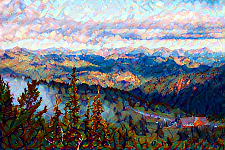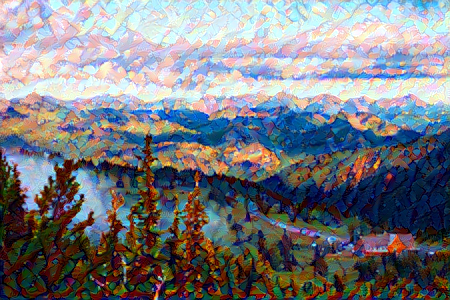# 9.11. 样式迁移¶## 9.11.1. 方法¶In :

%matplotlib inline
import d2lzh as d2l
from mxnet import autograd, gluon, image, init, nd
from mxnet.gluon import model_zoo, nn
import time

/var/lib/jenkins/miniconda3/envs/d2l-zh-build/lib/python3.6/site-packages/requests/__init__.py:91: RequestsDependencyWarning: urllib3 (1.25.11) or chardet (3.0.4) doesn't match a supported version!
RequestsDependencyWarning)


## 9.11.2. 读取内容图像和样式图像¶

In :

d2l.set_figsize()
d2l.plt.imshow(content_img.asnumpy());In :

style_img = image.imread('../img/autumn_oak.jpg')
d2l.plt.imshow(style_img.asnumpy());## 9.11.3. 预处理和后处理图像¶

In :

rgb_mean = nd.array([0.485, 0.456, 0.406])
rgb_std = nd.array([0.229, 0.224, 0.225])

def preprocess(img, image_shape):
img = image.imresize(img, *image_shape)
img = (img.astype('float32') / 255 - rgb_mean) / rgb_std
return img.transpose((2, 0, 1)).expand_dims(axis=0)

def postprocess(img):
img = img.as_in_context(rgb_std.context)
return (img.transpose((1, 2, 0)) * rgb_std + rgb_mean).clip(0, 1)


## 9.11.4. 抽取特征¶

In :

pretrained_net = model_zoo.vision.vgg19(pretrained=True)


In :

style_layers, content_layers = [0, 5, 10, 19, 28], 


In :

net = nn.Sequential()
for i in range(max(content_layers + style_layers) + 1):


In :

def extract_features(X, content_layers, style_layers):
contents = []
styles = []
for i in range(len(net)):
X = net[i](X)
if i in style_layers:
styles.append(X)
if i in content_layers:
contents.append(X)
return contents, styles


In :

def get_contents(image_shape, ctx):
content_X = preprocess(content_img, image_shape).copyto(ctx)
contents_Y, _ = extract_features(content_X, content_layers, style_layers)
return content_X, contents_Y

def get_styles(image_shape, ctx):
style_X = preprocess(style_img, image_shape).copyto(ctx)
_, styles_Y = extract_features(style_X, content_layers, style_layers)
return style_X, styles_Y


## 9.11.5. 定义损失函数¶

### 9.11.5.1. 内容损失¶

In :

def content_loss(Y_hat, Y):
return (Y_hat - Y).square().mean()


### 9.11.5.2. 样式损失¶

In :

def gram(X):
num_channels, n = X.shape, X.size // X.shape
X = X.reshape((num_channels, n))
return nd.dot(X, X.T) / (num_channels * n)


In :

def style_loss(Y_hat, gram_Y):
return (gram(Y_hat) - gram_Y).square().mean()


### 9.11.5.3. 总变差损失¶

$\sum_{i,j} \left|x_{i,j} - x_{i+1,j}\right| + \left|x_{i,j} - x_{i,j+1}\right|$

In :

def tv_loss(Y_hat):
return 0.5 * ((Y_hat[:, :, 1:, :] - Y_hat[:, :, :-1, :]).abs().mean() +
(Y_hat[:, :, :, 1:] - Y_hat[:, :, :, :-1]).abs().mean())


### 9.11.5.4. 损失函数¶

In :

content_weight, style_weight, tv_weight = 1, 1e3, 10

def compute_loss(X, contents_Y_hat, styles_Y_hat, contents_Y, styles_Y_gram):
# 分别计算内容损失、样式损失和总变差损失
contents_l = [content_loss(Y_hat, Y) * content_weight for Y_hat, Y in zip(
contents_Y_hat, contents_Y)]
styles_l = [style_loss(Y_hat, Y) * style_weight for Y_hat, Y in zip(
styles_Y_hat, styles_Y_gram)]
tv_l = tv_loss(X) * tv_weight
# 对所有损失求和
return contents_l, styles_l, tv_l, l


## 9.11.6. 创建和初始化合成图像¶

In :

class GeneratedImage(nn.Block):
def __init__(self, img_shape, **kwargs):
super(GeneratedImage, self).__init__(**kwargs)
self.weight = self.params.get('weight', shape=img_shape)

def forward(self):
return self.weight.data()


In :

def get_inits(X, ctx, lr, styles_Y):
gen_img = GeneratedImage(X.shape)
gen_img.initialize(init.Constant(X), ctx=ctx, force_reinit=True)
{'learning_rate': lr})
styles_Y_gram = [gram(Y) for Y in styles_Y]
return gen_img(), styles_Y_gram, trainer


## 9.11.7. 训练模型¶

In :

def train(X, contents_Y, styles_Y, ctx, lr, max_epochs, lr_decay_epoch):
X, styles_Y_gram, trainer = get_inits(X, ctx, lr, styles_Y)
for i in range(max_epochs):
start = time.time()
contents_Y_hat, styles_Y_hat = extract_features(
X, content_layers, style_layers)
contents_l, styles_l, tv_l, l = compute_loss(
X, contents_Y_hat, styles_Y_hat, contents_Y, styles_Y_gram)
l.backward()
trainer.step(1)
nd.waitall()
if i % 50 == 0 and i != 0:
print('epoch %3d, content loss %.2f, style loss %.2f, '
'TV loss %.2f, %.2f sec'
time.time() - start))
if i % lr_decay_epoch == 0 and i != 0:
trainer.set_learning_rate(trainer.learning_rate * 0.1)
print('change lr to %.1e' % trainer.learning_rate)
return X


In :

ctx, image_shape = d2l.try_gpu(), (225, 150)
net.collect_params().reset_ctx(ctx)
content_X, contents_Y = get_contents(image_shape, ctx)
_, styles_Y = get_styles(image_shape, ctx)
output = train(content_X, contents_Y, styles_Y, ctx, 0.01, 500, 200)

epoch  50, content loss 10.11, style loss 29.40, TV loss 3.46, 0.01 sec
epoch 100, content loss 7.50, style loss 15.46, TV loss 3.89, 0.01 sec
epoch 150, content loss 6.31, style loss 10.38, TV loss 4.15, 0.01 sec
epoch 200, content loss 5.64, style loss 8.11, TV loss 4.29, 0.01 sec
change lr to 1.0e-03
epoch 250, content loss 5.57, style loss 7.94, TV loss 4.30, 0.01 sec
epoch 300, content loss 5.52, style loss 7.79, TV loss 4.31, 0.01 sec
epoch 350, content loss 5.46, style loss 7.64, TV loss 4.31, 0.02 sec
epoch 400, content loss 5.40, style loss 7.49, TV loss 4.32, 0.01 sec
change lr to 1.0e-04
epoch 450, content loss 5.40, style loss 7.47, TV loss 4.32, 0.01 sec


In :

d2l.plt.imsave('../img/neural-style-1.png', postprocess(output).asnumpy())In :

image_shape = (450, 300)
_, content_Y = get_contents(image_shape, ctx)
_, style_Y = get_styles(image_shape, ctx)
X = preprocess(postprocess(output) * 255, image_shape)
output = train(X, content_Y, style_Y, ctx, 0.01, 300, 100)
d2l.plt.imsave('../img/neural-style-2.png', postprocess(output).asnumpy())

epoch  50, content loss 13.83, style loss 13.74, TV loss 2.38, 0.02 sec
epoch 100, content loss 9.56, style loss 8.77, TV loss 2.65, 0.02 sec
change lr to 1.0e-03
epoch 150, content loss 9.25, style loss 8.44, TV loss 2.68, 0.02 sec
epoch 200, content loss 8.98, style loss 8.15, TV loss 2.70, 0.02 sec
change lr to 1.0e-04
epoch 250, content loss 8.94, style loss 8.12, TV loss 2.70, 0.02 sec## 9.11.8. 小结¶

• 样式迁移常用的损失函数由3部分组成：内容损失使合成图像与内容图像在内容特征上接近，样式损失令合成图像与样式图像在样式特征上接近，而总变差损失则有助于减少合成图像中的噪点。
• 可以通过预训练的卷积神经网络来抽取图像的特征，并通过最小化损失函数来不断更新合成图像。
• 用格拉姆矩阵表达样式层输出的样式。

## 9.11.9. 练习¶

• 选择不同的内容和样式层，输出有什么变化？
• 调整损失函数中的权值超参数，输出是否保留更多内容或减少更多噪点？
• 替换实验中的内容图像和样式图像，你能创作出更有趣的合成图像吗？

## 9.11.10. 参考文献¶

 Gatys, L. A., Ecker, A. S., & Bethge, M. (2016). Image style transfer using convolutional neural networks. In Proceedings of the IEEE Conference on Computer Vision and Pattern Recognition (pp. 2414-2423).

## 9.11.11. 扫码直达讨论区¶